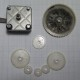## How to sketch equation curves in Fusion 360

The ability to sketch an equation curve is available in Autodesk’s more professional (and expensive) CAD product, Autodesk Inventor, but is missing from Fusion360’s. This tutorial details how you can get similar functionality using the Fusion 360 API.

The Fusion 360 API (Application Programming Interface) allows you to control the functionality of Fusion 360 by wiring code rather than directly through the graphical interface. This is a powerful tool which is useful for automating repetitive tasks and writing add-ins like those you will find on the Autodesk app-store.

In this tutorial we will be using the API in Fusion 360 to sketch a curve using an equation in both 2D and 3D. This is useful when you need to produce a specific, mathematically correct curve that can not be formed easily from primitive geometric shapes. For example, how would you sketch a line along the following equationwhich when plotted over range 0 to 6.3 (2pi) (spreadsheet here) looks like the following:To do so we can follow a few simple steps:

1. Get acquainted with the basics of how to use the API by watching the following short video from the Autodesk Design Academy channel.

2. Refer to the following example python script to suit your need which can be found here.

def run(context):

ui = None

try:

ui = app.userInterface

design = app.activeProduct

# Get the root component of the active design.

rootComp = design.rootComponent

# Create a new sketch on the xy plane.

sketches = rootComp.sketches

xyPlane = rootComp.xYConstructionPlane

points = adsk.core.ObjectCollection.create() # Create an object collection for the points.

# Enter variables here. E.g. E = 50

startRange = 0 # Start of range to be evaluated.

endRange = 2*math.pi # End of range to be evaluated.

splinePoints = 100 # Number of points that splines are generated.

# WARMING: Using more than a few hundred points may cause your system to hang.

i = 0

while i <= splinePoints:

t = startRange + ((endRange – startRange)/splinePoints)*i

xCoord = (math.sin(2*t))

yCoord = (math.sin(3*t))

i = i + 1

#Generates the spline curve

# Error handeling

except:

if ui:

ui.messageBox(‘Failed:\n{}’.format(traceback.format_exc()))

3. Either hit run from Anaconda (The python compiler installed by Fusion 360) or save the script and run it in Fusion 360 directly using the ‘Add-ins’ dialog box. The above script generates the following curve.To extrude the shape you can select the curve and use the ‘Open/Close Spline Curve’ function to form a closed sketch.Increasing the ‘splinePoints’ variable will increase the number of spline points generated and in-turn will produce a curve that more accurately fits the equation used. Below is a the same curve with 500 spline points.However, using a large number of spline points (approx. >500) may cause a considerable delay in generating your sketch or may even crash Fusion 360 completely.

Also note that the API exclusively works in the units of cm for length, radians for angles (radians = degrees * pi/180) and kg for mass. If you prefer to work in different units see this section of the Fusion 360 API manual.

To extend this concept into 3D space we only need to add another coordinate Z and include that in the points object.

while i <= splinePoints:

t = startRange + ((endRange – startRange)/splinePoints)*i

xCoord = (math.sin(2*t))

yCoord = (math.sin(3*t))

zCoord = 2**t

i = i + 1

#Generates the spline curve

Below is how this sketch looks when viewed face on and at an angle.That’s it for this post. For a more in-depth understanding of the API be sure to take a look at the Fusion 360 API User manual, sample scripts and the API training series playlist on YouTube.

Big thanks to Macaba on the Odrive discord channel for showing me his cycloidal script  which I modified to use as the example in this post.I am a Materials Engineering working in the field of Magnetic Materials in Melbourne, Australia. This blog covers my personal interest in all things CNC.
This entry was posted in 3D Design and tagged , , , , . Bookmark the permalink.

### 3 Responses to How to sketch equation curves in Fusion 360

1.Luigi says:

amazing!

2.Luigi says:

can I use pycharm and import it into the fusion 360 ?

•neuman1001 says:

Autodesk Fusion 360 automatically used code since it’s setup as my .py editor, so I’m sure you could use pycharm as well.